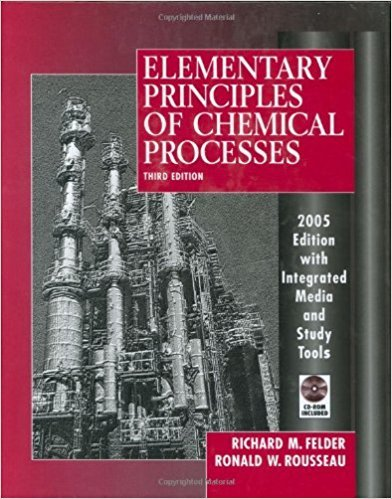×
Get Full Access to Elementary Principles Of Chemical Processes - 3 Edition - Chapter 7 - Problem 7.29
Get Full Access to Elementary Principles Of Chemical Processes - 3 Edition - Chapter 7 - Problem 7.29

×

# Superheated steam at 40 bar absolute and sooac flows at aISBN: 9780471687573 143

## Solution for problem 7.29 Chapter 7

Elementary Principles of Chemical Processes | 3rd Edition

• Textbook Solutions
• 2901 Step-by-step solutions solved by professors and subject experts
• Get 24/7 help from StudySoup virtual teaching assistantsElementary Principles of Chemical Processes | 3rd Edition

4 5 1 324 Reviews
24
2
Problem 7.29

Superheated steam at 40 bar absolute and sooac flows at a rate of 250 kg/min to an adiabatic turbine, where it expands to 5 bar. The turbine develops 1500 kW. From the turbine the steam flows to a heater, where it is reheated isobarically to its initial temperature. Neglect kinetic energy changes. (a) Write an energy balance on the turbine and use it to determine the outlet stream temperature. (b) Write an energy balance on the heater and use it to determine the required input (kW) to the steam. (c) Verify that an overall energy balance on the two-unit process is satisfied. (d) Suppose the turbine inlet and outlet pipes both have diameters of 0.5 meter. Show that it is reasonable to neglect the change in kinetic energy for this unit.

Step-by-Step Solution:
Step 1 of 3

|r--c\-* re t+-e-H oll Eva'ts C'Cl.^n H.b ;1042 o b p F.jTV+" bW ^ppLcs br ''N€ul.-rPAUFa"n k elet+'b^s&i) rl rl 1 7't :l ( 96'2 t-3t+ & L. conncch t+ t- b^oJ,r-€)e.h"n-r: F,L. -=t - Ll -o i t\'- A/'-a\H

Step 2 of 3

Step 3 of 3

##### ISBN: 9780471687573

The answer to “Superheated steam at 40 bar absolute and sooac flows at a rate of 250 kg/min to an adiabatic turbine, where it expands to 5 bar. The turbine develops 1500 kW. From the turbine the steam flows to a heater, where it is reheated isobarically to its initial temperature. Neglect kinetic energy changes. (a) Write an energy balance on the turbine and use it to determine the outlet stream temperature. (b) Write an energy balance on the heater and use it to determine the required input (kW) to the steam. (c) Verify that an overall energy balance on the two-unit process is satisfied. (d) Suppose the turbine inlet and outlet pipes both have diameters of 0.5 meter. Show that it is reasonable to neglect the change in kinetic energy for this unit.” is broken down into a number of easy to follow steps, and 131 words. The full step-by-step solution to problem: 7.29 from chapter: 7 was answered by , our top Chemistry solution expert on 11/15/17, 02:42PM. Since the solution to 7.29 from 7 chapter was answered, more than 310 students have viewed the full step-by-step answer. This full solution covers the following key subjects: turbine, Energy, steam, balance, determine. This expansive textbook survival guide covers 13 chapters, and 710 solutions. Elementary Principles of Chemical Processes was written by and is associated to the ISBN: 9780471687573. This textbook survival guide was created for the textbook: Elementary Principles of Chemical Processes, edition: 3.

Unlock Textbook Solution